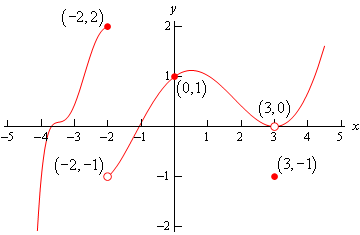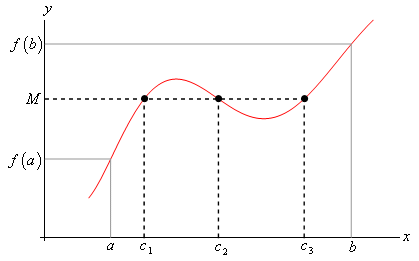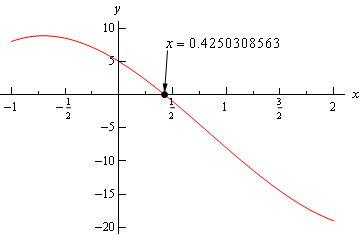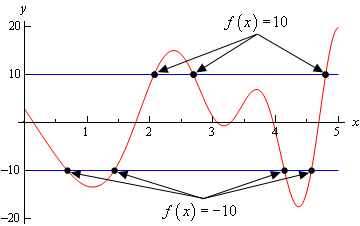Paul's Online Notes
Home / Calculus I / Limits / Continuity
Show Mobile Notice Show All Notes Hide All Notes
Mobile Notice
You appear to be on a device with a "narrow" screen width (i.e. you are probably on a mobile phone). Due to the nature of the mathematics on this site it is best views in landscape mode. If your device is not in landscape mode many of the equations will run off the side of your device (should be able to scroll to see them) and some of the menu items will be cut off due to the narrow screen width.

### Section 2-9 : Continuity

Over the last few sections we’ve been using the term “nice enough” to define those functions that we could evaluate limits by just evaluating the function at the point in question. It’s now time to formally define what we mean by “nice enough”.

#### Definition

A function $$f\left( x \right)$$ is said to be continuous at $$x = a$$ if

$\mathop {\lim }\limits_{x \to a} f\left( x \right) = f\left( a \right)$

A function is said to be continuous on the interval $$\left[ {a,b} \right]$$ if it is continuous at each point in the interval.

Note that this definition is also implicitly assuming that both $$f\left( a \right)$$ and $$\mathop {\lim }\limits_{x \to a} f\left( x \right)$$ exist. If either of these do not exist the function will not be continuous at $$x = a$$.

This definition can be turned around into the following fact.

#### Fact I

If $$f\left( x \right)$$ is continuous at $$x = a$$ then,

$\mathop {\lim }\limits_{x \to a} f\left( x \right) = f\left( a \right)\hspace{0.5in}\mathop {\lim }\limits_{x \to {a^ - }} f\left( x \right) = f\left( a \right)\hspace{0.5in}\mathop {\lim }\limits_{x \to {a^ + }} f\left( x \right) = f\left( a \right)$

This is exactly the same fact that we first put down back when we started looking at limits with the exception that we have replaced the phrase “nice enough” with continuous.

It’s nice to finally know what we mean by “nice enough”, however, the definition doesn’t really tell us just what it means for a function to be continuous. Let’s take a look at an example to help us understand just what it means for a function to be continuous.

Example 1 Given the graph of $$f\left( x \right)$$, shown below, determine if $$f\left( x \right)$$ is continuous at $$x = - 2$$, $$x = 0$$, and $$x = 3$$.Show Solution

To answer the question for each point we’ll need to get both the limit at that point and the function value at that point. If they are equal the function is continuous at that point and if they aren’t equal the function isn’t continuous at that point.

First $$x = - 2$$.

$f\left( { - 2} \right) = 2\hspace{0.5in}\mathop {\lim }\limits_{x \to - 2} f\left( x \right)\,\,\,{\mbox{doesn't exist}}$

The function value and the limit aren’t the same and so the function is not continuous at this point. This kind of discontinuity in a graph is called a jump discontinuity. Jump discontinuities occur where the graph has a break in it as this graph does and the values of the function to either side of the break are finite (i.e. the function doesn’t go to infinity).

Now $$x = 0$$.

$f\left( 0 \right) = 1\hspace{0.5in}\mathop {\lim }\limits_{x \to 0} f\left( x \right) = 1$

The function is continuous at this point since the function and limit have the same value.

Finally $$x = 3$$.

$f\left( 3 \right) = - 1\hspace{0.5in}\mathop {\lim }\limits_{x \to 3} f\left( x \right) = 0$

The function is not continuous at this point. This kind of discontinuity is called a removable discontinuity. Removable discontinuities are those where there is a hole in the graph as there is in this case.

From this example we can get a quick “working” definition of continuity. A function is continuous on an interval if we can draw the graph from start to finish without ever once picking up our pencil. The graph in the last example has only two discontinuities since there are only two places where we would have to pick up our pencil in sketching it.

In other words, a function is continuous if its graph has no holes or breaks in it.

For many functions it’s easy to determine where it won’t be continuous. Functions won’t be continuous where we have things like division by zero or logarithms of zero. Let’s take a quick look at an example of determining where a function is not continuous.

Example 2 Determine where the function below is not continuous. $h\left( t \right) = \frac{{4t + 10}}{{{t^2} - 2t - 15}}$
Show Solution

Rational functions are continuous everywhere except where we have division by zero. So all that we need to is determine where the denominator is zero. That’s easy enough to determine by setting the denominator equal to zero and solving.

${t^2} - 2t - 15 = \left( {t - 5} \right)\left( {t + 3} \right) = 0$

So, the function will not be continuous at $$t=-3$$ and $$t=5$$.

A nice consequence of continuity is the following fact.

#### Fact 2

If $$f\left( x \right)$$ is continuous at $$x = b$$ and $$\mathop {\lim }\limits_{x \to a} g\left( x \right) = b$$ then,

$\mathop {\lim }\limits_{x \to a} f\left( {g\left( x \right)} \right) = f\left( {\mathop {\lim }\limits_{x \to a} g\left( x \right)} \right)$

To see a proof of this fact see the Proof of Various Limit Properties section in the Extras chapter. With this fact we can now do limits like the following example.

Example 3 Evaluate the following limit. $\mathop {\lim }\limits_{x \to 0} {{\bf{e}}^{\sin x}}$
Show Solution

Since we know that exponentials are continuous everywhere we can use the fact above.

$\mathop {\lim }\limits_{x \to 0} {{\bf{e}}^{\sin x}} = {{\bf{e}}^{\mathop {\lim }\limits_{x \to 0} \sin x}} = {{\bf{e}}^0} = 1$

Another very nice consequence of continuity is the Intermediate Value Theorem.

#### Intermediate Value Theorem

Suppose that $$f\left( x \right)$$ is continuous on $$\left[ {a,b} \right]$$ and let $$M$$ be any number between $$f\left( a \right)$$ and $$f\left( b \right)$$. Then there exists a number $$c$$ such that,

1. $$a < c < b$$
2. $$f\left( c \right) = M$$

All the Intermediate Value Theorem is really saying is that a continuous function will take on all values between $$f\left( a \right)$$ and $$f\left( b \right)$$. Below is a graph of a continuous function that illustrates the Intermediate Value Theorem.As we can see from this image if we pick any value, $$M$$, that is between the value of $$f\left( a \right)$$ and the value of $$f\left( b \right)$$ and draw a line straight out from this point the line will hit the graph in at least one point. In other words, somewhere between $$a$$ and $$b$$ the function will take on the value of $$M$$. Also, as the figure shows the function may take on the value at more than one place.

It’s also important to note that the Intermediate Value Theorem only says that the function will take on the value of $$M$$ somewhere between $$a$$ and $$b$$. It doesn’t say just what that value will be. It only says that it exists.

So, the Intermediate Value Theorem tells us that a function will take the value of $$M$$ somewhere between $$a$$ and $$b$$ but it doesn’t tell us where it will take the value nor does it tell us how many times it will take the value. These are important ideas to remember about the Intermediate Value Theorem.

A nice use of the Intermediate Value Theorem is to prove the existence of roots of equations as the following example shows.

Example 4 Show that $$p\left( x \right) = 2{x^3} - 5{x^2} - 10x + 5$$ has a root somewhere in the interval $$\left[ { - 1,2} \right]$$.
Show Solution

What we’re really asking here is whether or not the function will take on the value

$p\left( x \right) = 0$

somewhere between -1 and 2. In other words, we want to show that there is a number $$c$$ such that $$- 1 < c < 2$$ and $$p\left( c \right) = 0$$. However if we define $$M = 0$$ and acknowledge that $$a = - 1$$ and $$b = 2$$ we can see that these two condition on $$c$$ are exactly the conclusions of the Intermediate Value Theorem.

So, this problem is set up to use the Intermediate Value Theorem and in fact, all we need to do is to show that the function is continuous and that $$M = 0$$ is between $$p\left( { - 1} \right)$$ and $$p\left( 2 \right)$$ (i.e. $$p\left( { - 1} \right) < 0 < p\left( 2 \right)$$ or $$p\left( 2 \right) < 0 < p\left( { - 1} \right)$$ and we’ll be done.

To do this all we need to do is compute,

$p\left( { - 1} \right) = 8\hspace{0.5in}\hspace{0.25in}p\left( 2 \right) = - 19$

So, we have,

$- 19 = p\left( 2 \right) < 0 < p\left( { - 1} \right) = 8$

Therefore $$M = 0$$ is between $$p\left( { - 1} \right)$$ and $$p\left( 2 \right)$$ and since $$p\left( x \right)$$ is a polynomial it’s continuous everywhere and so in particular it’s continuous on the interval $$[-1,2]$$. So by the Intermediate Value Theorem there must be a number $$- 1 < c < 2$$ so that,

$p\left( c \right) = 0$

Therefore, the polynomial does have a root between -1 and 2.

For the sake of completeness here is a graph showing the root that we just proved existed. Note that we used a computer program to actually find the root and that the Intermediate Value Theorem did not tell us what this value was.Let’s take a look at another example of the Intermediate Value Theorem.

Example 5 If possible, determine if $$f\left( x \right) = 20\sin \left( {x + 3} \right)\cos \left( {\frac{{{x^2}}}{2}} \right)$$ takes the following values in the interval $$[0,5]$$.
1. Does $$f\left( x \right) = 10$$?
2. Does $$f\left( x \right) = - 10$$?
Show All Solutions Hide All Solutions
Show Discussion

Okay, so as with the previous example, we’re being asked to determine, if possible, if the function takes on either of the two values above in the interval [0,5]. First, let’s notice that this is a continuous function and so we know that we can use the Intermediate Value Theorem to do this problem.

Now, for each part we will let $$M$$ be the given value for that part and then we’ll need to show that $$M$$ lives between $$f\left( 0 \right)$$ and $$f\left( 5 \right)$$. If it does, then we can use the Intermediate Value Theorem to prove that the function will take the given value.

So, since we’ll need the two function evaluations for each part let’s give them here,

$f\left( 0 \right) = 2.8224\hspace{0.5in}\hspace{0.25in}f\left( 5 \right) = 19.7436$

Now, let’s take a look at each part.

a Does $$f\left( x \right) = 10$$? Show Solution

Okay, in this case we’ll define $$M = 10$$ and we can see that,

$f\left( 0 \right) = 2.8224 < 10 < 19.7436 = f\left( 5 \right)$

So, by the Intermediate Value Theorem there must be a number $$0 \le c \le 5$$ such that

$f\left( c \right) = 10$

b Does $$f\left( x \right) = - 10$$? Show Solution

In this part we’ll define $$M = - 10$$. We now have a problem. In this part $$M$$ does not live between $$f\left( 0 \right)$$ and $$f\left( 5 \right)$$. So, what does this mean for us? Does this mean that $$f\left( x \right) \ne - 10$$ in $$[0,5]$$?

Unfortunately for us, this doesn’t mean anything. It is possible that $$f\left( x \right) \ne - 10$$ in $$[0,5]$$, but is it also possible that $$f\left( x \right) = - 10$$ in $$[0,5]$$. The Intermediate Value Theorem will only tell us that $$c$$’s will exist. The theorem will NOT tell us that $$c$$’s don’t exist.

In this case it is not possible to determine if $$f\left( x \right) = - 10$$ in $$[0,5]$$ using the Intermediate Value Theorem.

Okay, as the previous example has shown, the Intermediate Value Theorem will not always be able to tell us what we want to know. Sometimes we can use it to verify that a function will take some value in a given interval and in other cases we won’t be able to use it.

For completeness sake here is the graph of $$f\left( x \right) = 20\sin \left( {x + 3} \right)\cos \left( {\frac{{{x^2}}}{2}} \right)$$ in the interval [0,5].From this graph we can see that not only does $$f\left( x \right) = - 10$$ in [0,5] it does so a total of 4 times! Also note that as we verified in the first part of the previous example $$f\left( x \right) = 10$$ in [0,5] and in fact it does so a total of 3 times.

So, remember that the Intermediate Value Theorem will only verify that a function will take on a given value. It will never exclude a value from being taken by the function. Also, if we can use the Intermediate Value Theorem to verify that a function will take on a value it never tells us how many times the function will take on the value, it only tells us that it does take the value.